# Hardest colleges to get into in Maine

Top 10 colleges in Maine with the lowest acceptance rates
What are the hardest colleges to get into in Maine? We've got you covered. We've compiled a national college database and have created a list of the hardest universities to get into in Maine below. These are the hardest 4 year colleges to get into in Maine, and sometimes some of the hardest colleges to get into in the US. You could even say these are the best colleges in Maine. We also include each college's average SAT and ACT scores so that you can see where you're most competitive. Read on to find out more.

## Bowdoin College acceptance rate

Bowdoin College acceptance rate is 10.3%.The average SAT score for Bowdoin College is 1405.

• SAT composite: 1405
• SAT math: 710

The average ACT score for Bowdoin College is 32.

## Colby College acceptance rate

Colby College acceptance rate is 13.1%.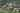The average SAT score for Colby College is 1430.

• SAT composite: 1430
• SAT math: 730

The average ACT score for Colby College is 32.

## Bates College acceptance rate

Bates College acceptance rate is 17.8%.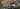The average SAT score for Bates College is 1380.

• SAT composite: 1380
• SAT math: 700

The average ACT score for Bates College is 31.

## College of the Atlantic acceptance rate

College of the Atlantic acceptance rate is 67.9%.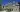The average SAT score for College of the Atlantic is 1269.

• SAT composite: 1269
• SAT math: 600

The average ACT score for College of the Atlantic is 28.

## Maine Maritime Academy acceptance rate

Maine Maritime Academy acceptance rate is 78.6%.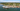The average SAT score for Maine Maritime Academy is 1110.

• SAT composite: 1110
• SAT math: 560

The average ACT score for Maine Maritime Academy is 22.

## Husson University acceptance rate

Husson University acceptance rate is 80.2%.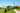The average SAT score for Husson University is 1060.

• SAT composite: 1060
• SAT math: 520

The average ACT score for Husson University is 20.

## University of New England acceptance rate

University of New England acceptance rate is 80.7%.The average SAT score for University of New England is 1127.

• SAT composite: 1127
• SAT math: 560

The average ACT score for University of New England is 24.

## Saint Joseph's College of Maine acceptance rate

Saint Joseph's College of Maine acceptance rate is 88.1%.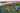The average SAT score for Saint Joseph's College of Maine is 1040.

• SAT composite: 1040
• SAT math: 510

The average ACT score for Saint Joseph's College of Maine is 22.

## Unity College acceptance rate

Unity College acceptance rate is 88.7%.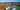The average SAT score for Unity College is 1100.

• SAT composite: 1100
• SAT math: 540

The average ACT score for Unity College is 22.

## University of Maine at Presque Isle acceptance rate

University of Maine at Presque Isle acceptance rate is 91.6%.The average SAT score for University of Maine at Presque Isle is 998.

• SAT composite: 998
• SAT math: 490